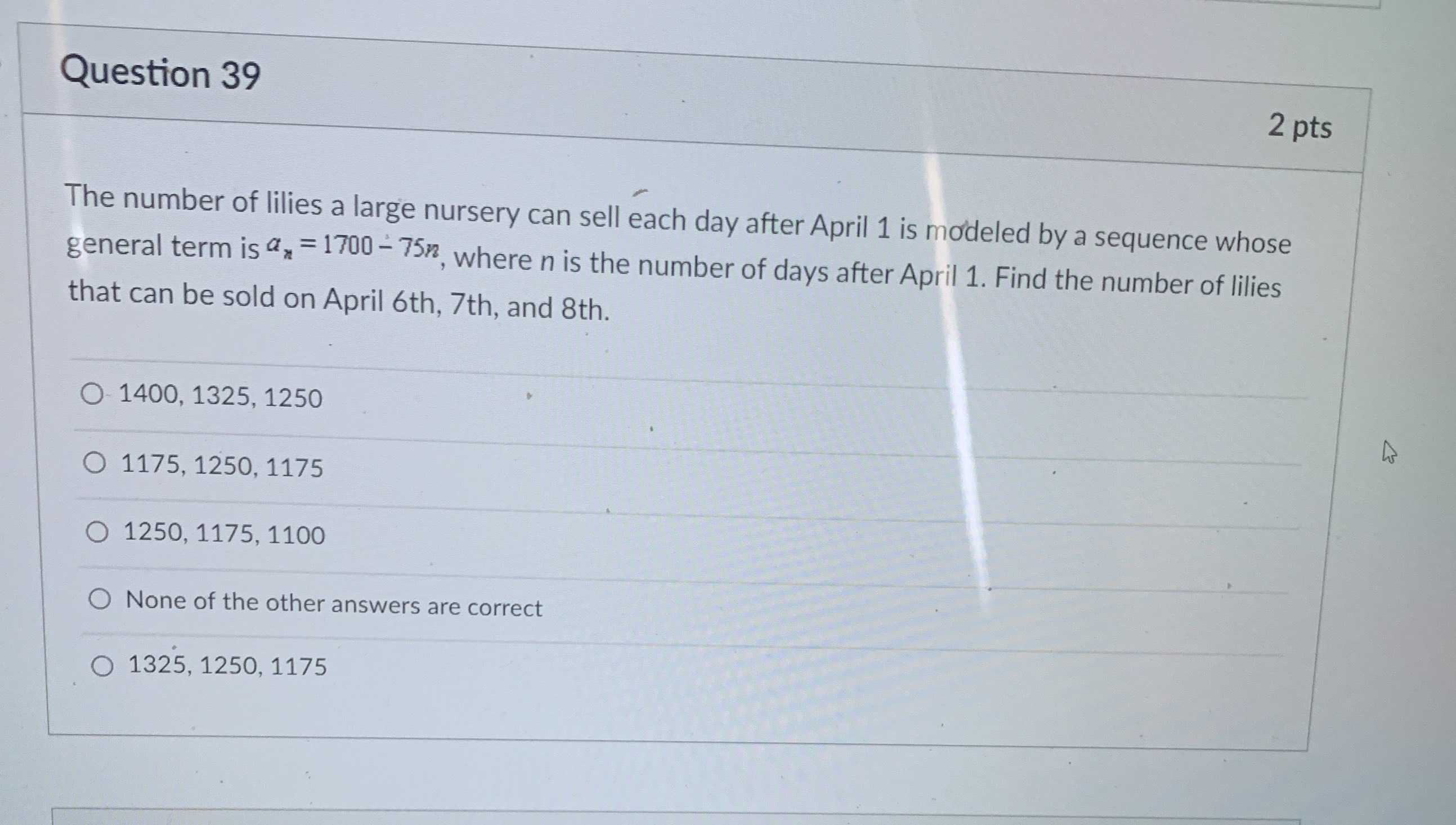### Still have math questions?

Algebra
QuestionThe number of lilies a large nursery can sell each day after April $$1$$ is mơdeled by a sequence whose general term is $$a _ { n } = 1700 - 75 n$$ , where $$n$$ is the number of days after April $$1 .$$ Find the number of lilies that can be sold on April $$6$$ th, $$7$$ th, and $$8$$ th.

1400, $$1325,1250$$

$$1175,1250,1175$$

$$1250,1175,1100$$

None of the other answers are correct

$$1325,1250,1175$$